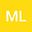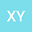Time periodic solutions for the full quantum Euler equation
••• Min LI,
• Xianzhong Yao
Min LI
Shanxi University of Finance and Economics
Author ProfileXianzhong Yao
Shanxi University of Finance and Economics
Author Profile## Abstract

In this paper, we establish the existence and uniqueness of a time periodic solution to the full compressible quantum Euler equations. First, we prove the existence of time periodic solutions under some smallness assumptions imposed on the external force in a periodic domain by a regularized approximation scheme and the Leray-Schauder degree theory. Then the result is generalized to $\mathbb{R}^{3}$ by adapting a limiting method and a diagonal argument. The uniqueness of the time periodic solutions is also given. Compared to classical Euler equations, the third-order quantum spatial derivatives are considered which need some elaborated treatments thereof in obtaining the highest-order energy estimates.

#### Peer review status:POSTED

03 Aug 2020Submitted to Mathematical Methods in the Applied Sciences
04 Aug 2020Assigned to Editor
04 Aug 2020Submission Checks Completed